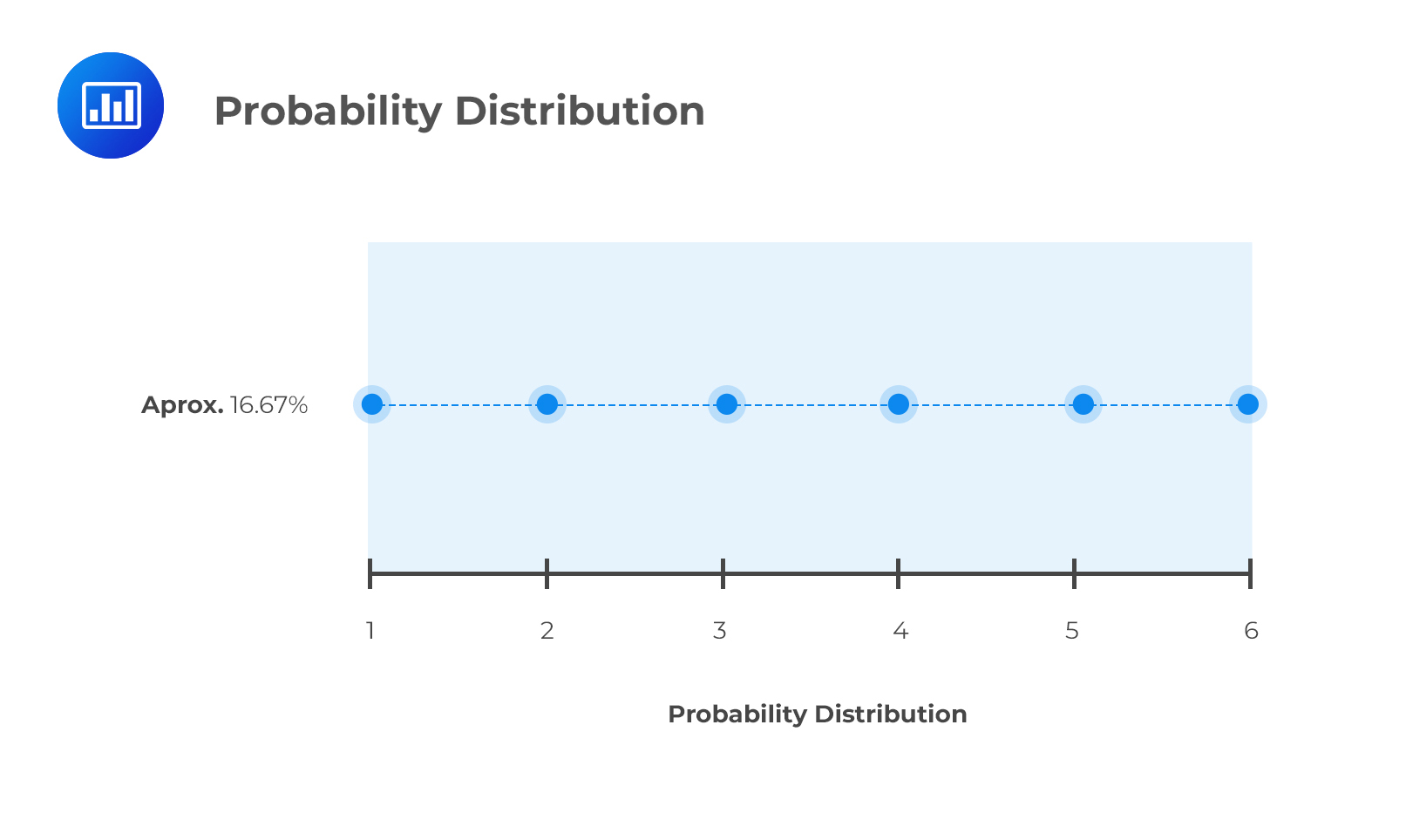Limited Time Offer: Save 10% on all 2021 and 2022 Premium Study Packages with promo code: BLOG10    Select your Premium Package »Introduction to Probability Distributions, Probability Functions, and Types of Variables

Probability Distribution

The probability distribution of a random variable “X” is basically a graphical presentation of the probabilities of all possible outcomes of X. A random variable is any quantity for which more than one value is possible. An example of a random variable is the price of quoted stocks. Simply put, a probability distribution gathers all the outcomes and goes a step further to indicate the probability associated with each outcome.

What if we rolled a six-sided dice? The set of possible outcomes is:

{1   2   3   4   5   6}

Each of these outcomes would occur with a probability of 1/6 because each of them has an equal chance of occurring. Consequently, the probability distribution would be a straight line:Note to candidates: although the above distribution is a straight line, most real life distributions are usually curved. The CFA curriculum particularly delves into the bell-shaped normal distribution.

Discrete Random Variables

A discrete random variable can take on a finite number of outcomes.

Examples

1. If we roll a dice, there are 6 possible outcomes. Therefore, the outcomes are discrete and random.
2. The number of CFA finalists employed within a given year is a discrete random variable.

Continuous Random Variables

A continuous random variable is that which has an infinite number of possible outcomes. A good example can be the rate of return on a stock. For instance, the return can be 6% or between 6% and 7%, in which case it can take on 6.4%, 6.41%, 6.412%, or even 6.412325%, i.e., infinite values.

Probability Function

A probability function gives the probability of a random variable X taking on a value “x.” The probability functions of discrete and continuous random variables are slightly different.

For a discrete random variable, the probability function, P(x), satisfies the properties below.

1. P(X = x) = P(x)
In statistics, P(x) is said to be a probability mass function
2. P(x) is always nonnegative for all x
3. The sum of the probabilities of all possible outcomes = 1.

For a continuous random variable, the probability function, f(x), satisfies the properties listed below.

1. The probability that x is between two values, a and b is
$$P\left\{ a\le x\le b \right\} =\int _{ a }^{ b }{ f\left( x \right) dx }$$
f(x) is said to be a probability density function(pdf)
2. f(x) is nonnegative for all x
3. The integral of the probability function is 1, that is,
$$\int _{ -\infty }^{ \infty }{ f\left( x \right) dx=1 }$$

Define a probability distribution and distinguish between discrete and continuous random variables and their probability functions.

Featured Study with UsCFA® Exam and FRM® Exam Prep Platform offered by AnalystPrep

Study Platform

Learn with Us

Subscribe to our newsletter and keep up with the latest and greatest tips for success
Online TutoringOur videos feature professional educators presenting in-depth explanations of all topics introduced in the curriculum.

Video LessonsSergio Torrico
2021-07-23
Excelente para el FRM 2 Escribo esta revisión en español para los hispanohablantes, soy de Bolivia, y utilicé AnalystPrep para dudas y consultas sobre mi preparación para el FRM nivel 2 (lo tomé una sola vez y aprobé muy bien), siempre tuve un soporte claro, directo y rápido, el material sale rápido cuando hay cambios en el temario de GARP, y los ejercicios y exámenes son muy útiles para practicar.diana
2021-07-17
So helpful. I have been using the videos to prepare for the CFA Level II exam. The videos signpost the reading contents, explain the concepts and provide additional context for specific concepts. The fun light-hearted analogies are also a welcome break to some very dry content. I usually watch the videos before going into more in-depth reading and they are a good way to avoid being overwhelmed by the sheer volume of content when you look at the readings.Kriti Dhawan
2021-07-16
A great curriculum provider. James sir explains the concept so well that rather than memorising it, you tend to intuitively understand and absorb them. Thank you ! Grateful I saw this at the right time for my CFA prep.nikhil kumar
2021-06-28
Very well explained and gives a great insight about topics in a very short time. Glad to have found Professor Forjan's lectures.Marwan
2021-06-22
Great support throughout the course by the team, did not feel neglectedBenjamin anonymous
2021-05-10
I loved using AnalystPrep for FRM. QBank is huge, videos are great. Would recommend to a friendDaniel Glyn
2021-03-24
I have finished my FRM1 thanks to AnalystPrep. And now using AnalystPrep for my FRM2 preparation. Professor Forjan is brilliant. He gives such good explanations and analogies. And more than anything makes learning fun. A big thank you to Analystprep and Professor Forjan. 5 stars all the way!michael walshe
2021-03-18
Professor James' videos are excellent for understanding the underlying theories behind financial engineering / financial analysis. The AnalystPrep videos were better than any of the others that I searched through on YouTube for providing a clear explanation of some concepts, such as Portfolio theory, CAPM, and Arbitrage Pricing theory. Watching these cleared up many of the unclarities I had in my head. Highly recommended.